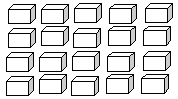Name: ___________________Date:___________________

 Email us to get an instant 20% discount on highly effective K-12 Math & English kwizNET Programs!

### Grade 3 - Mathematics1.18 Multiplication Review TestName: ___________________Date:___________________

### Grade 3 - Mathematics1.18 Multiplication Review Test

 Q 1: 11 x 11 =?121110120100 Q 2: Square of 8 is 8 x 8 =Answer: Q 3: 8 x 9 =Answer: Q 4: 12 x 12 =?122165142144 Q 5: 4x5=?2015510 Q 6: 6 x 8 = 48524536 Q 7: Use the figure to find 5 times 4.201921 Q 8: 11 X 1 =?110111 Question 9: This question is available to subscribers only! Question 10: This question is available to subscribers only!